# Masking and extrapolation in xESMF¶

(contributed by Raphael Dussin, based on previous work from jhamman, RondeauG, trondkr and others)

By default, xESMF treats NaNs like regular values hence potentially resulting in missing values bleeding into the regridded field and creating insconsistencies in the resulting masked array. To overcome this issue, we can use explicit masking of the source and target grids.

:

import xarray as xr
import xesmf
import numpy as np

:

import warnings

warnings.filterwarnings("ignore")


## Preparing the grids¶

For this tutorial, we’re using a dataset from the ROMS ocean model from this xarray tutorial

:

ds = xr.tutorial.open_dataset("ROMS_example.nc", chunks={"ocean_time": 1})


To use conservative regidding, we need the cells corners. Since they are not provided, we are creating some using a crude approximation. Please don’t try this at home!

:

lon_centers = ds["lon_rho"].values
lat_centers = ds["lat_rho"].values

lon_corners = 0.25 * (
lon_centers[:-1, :-1]
+ lon_centers[1:, :-1]
+ lon_centers[:-1, 1:]
+ lon_centers[1:, 1:]
)

lat_corners = 0.25 * (
lat_centers[:-1, :-1]
+ lat_centers[1:, :-1]
+ lat_centers[:-1, 1:]
+ lat_centers[1:, 1:]
)

ds["lon_psi"] = xr.DataArray(data=lon_corners, dims=("eta_psi", "xi_psi"))
ds["lat_psi"] = xr.DataArray(data=lat_corners, dims=("eta_psi", "xi_psi"))

ds = ds.assign_coords({"lon_psi": ds["lon_psi"], "lat_psi": ds["lat_psi"]})

# remove exterior rho points and cut 9 extra points to make
# zeta divisible by 10 for coarsening
ds = ds.isel(
eta_rho=slice(1, -10),
xi_rho=slice(1, -10),
eta_psi=slice(0, -9),
xi_psi=slice(0, -9),
)


We also need a coarse resolution grid. We’re going to build one by coarsening the ROMS dataset. coarsen.mean() typically works as a nan-mean on the 10x10 blocks of the grid so the resulting land mask looks like a flooded version of the original.

:

ds_coarse = xr.Dataset()

ds_coarse["zeta"] = xr.DataArray(
ds["zeta"].coarsen(xi_rho=10, eta_rho=10).mean().values,
dims=("ocean_time", "eta_rho", "xi_rho"),
)
# we want to subsample coordinates instead of coarsening them
ds_coarse["lon_rho"] = xr.DataArray(
ds["lon_rho"].values[::10, ::10], dims=("eta_rho", "xi_rho")
)
ds_coarse["lon_psi"] = xr.DataArray(
ds["lon_psi"].values[::10, ::10], dims=("eta_psi", "xi_psi")
)
ds_coarse["lat_rho"] = xr.DataArray(
ds["lat_rho"].values[::10, ::10], dims=("eta_rho", "xi_rho")
)
ds_coarse["lat_psi"] = xr.DataArray(
ds["lat_psi"].values[::10, ::10], dims=("eta_psi", "xi_psi")
)


We now have our 2 grids to test the masking in xESMF. Now let’s say we want to conservatively remap the fine ocean model output onto the coarse resolution grid.

:

ds["zeta"].isel(ocean_time=0).plot()

:

<matplotlib.collections.QuadMesh at 0x7f90e00919a0>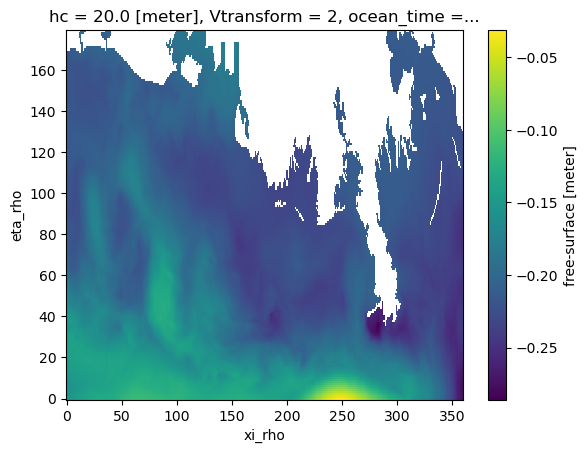:

ds_coarse["zeta"].isel(ocean_time=0).plot()

:

<matplotlib.collections.QuadMesh at 0x7f90c35355b0>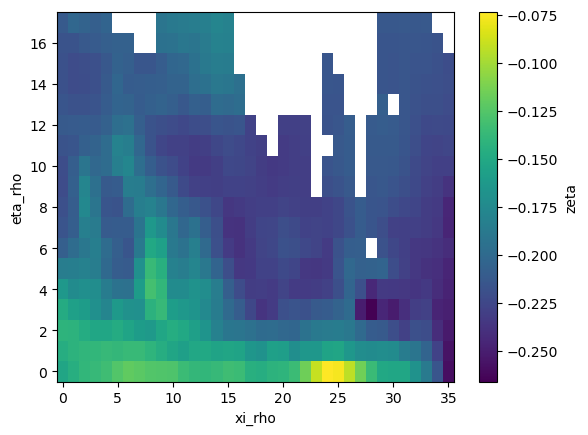As usual, xESMF expects fixed variable names for longitude/latitude in cell centers and corners:

:

ds["lon"] = ds["lon_rho"]
ds["lat"] = ds["lat_rho"]
ds["lon_b"] = ds["lon_psi"]
ds["lat_b"] = ds["lat_psi"]

ds_coarse["lon"] = ds_coarse["lon_rho"]
ds_coarse["lat"] = ds_coarse["lat_rho"]
ds_coarse["lon_b"] = ds_coarse["lon_psi"]
ds_coarse["lat_b"] = ds_coarse["lat_psi"]


In our first test, there is no masking involved and we define the regridder the typical way:

:

regrid_nomask = xesmf.Regridder(ds, ds_coarse, method="conservative_normed")

:

zeta_remapped = regrid_nomask(ds["zeta"])

:

zeta_remapped.isel(ocean_time=0).plot()

:

<matplotlib.collections.QuadMesh at 0x7f9148546e80>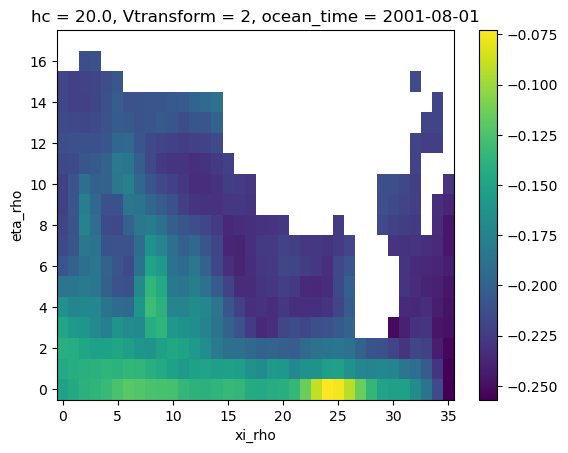Because of the missing values (NaNs) bleeding into the regridding, we end up with a land mask that is much bigger than the one of the coarse grid. That’s where masking is gonna help us getting it right.

To use masking, we need to add a dataarray named mask to our datasets. Let’s define our masks on the high and coarse resolution grids from the missing values in the zeta array:

:

ds["mask"] = xr.where(~np.isnan(ds["zeta"].isel(ocean_time=0)), 1, 0)

:

ds["mask"].plot(cmap="binary_r")

:

<matplotlib.collections.QuadMesh at 0x7f9148489100>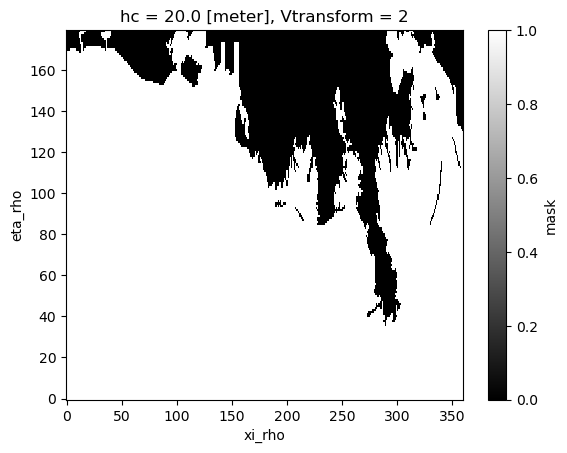:

ds_coarse["mask"] = xr.where(
~np.isnan(ds_coarse["zeta"].isel(ocean_time=0)), 1, 0
)

:

ds_coarse["mask"].plot(cmap="binary_r")

:

<matplotlib.collections.QuadMesh at 0x7f91483a3f40>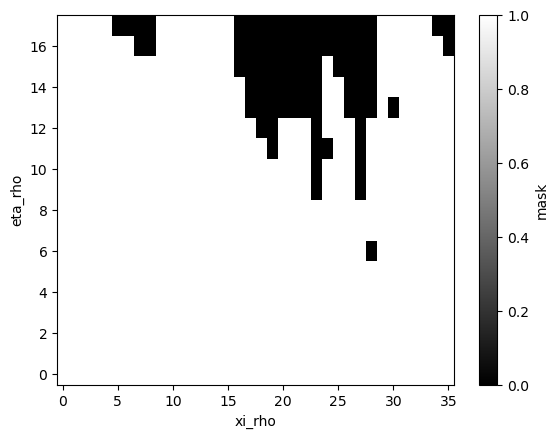Now let’s try to regrid again:

:

regrid_mask = xesmf.Regridder(ds, ds_coarse, method="conservative_normed")

:

zeta_remapped = regrid_mask(ds["zeta"])

:

zeta_remapped.isel(ocean_time=0).plot()

:

<matplotlib.collections.QuadMesh at 0x7f9136188640>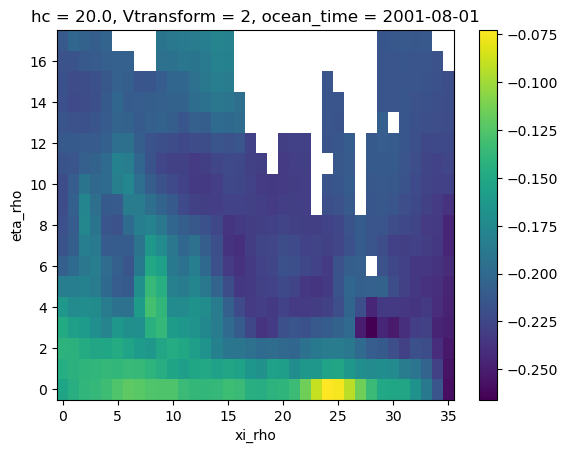Now we have our conservative remapping consistent with the coarse grid, yay!!

## Limitations and warnings¶

• mask can only be 2D (ESMF design) so regridding a 3D field requires to generate regridding weights for each vertical level.

• conservative method will give you a normalization by the total area of the target cell. Except for some specific cases, you probably want to use conservative_normed.

• results with other methods (e.g. bilinear) may not give masks consistent with the coarse grid.

### 1. Conservative (un-normed) example¶

:

regrid_masked2 = xesmf.Regridder(ds, ds_coarse, method="conservative")
zeta_remapped2.isel(ocean_time=0).plot()

:

<matplotlib.collections.QuadMesh at 0x7f91484142e0>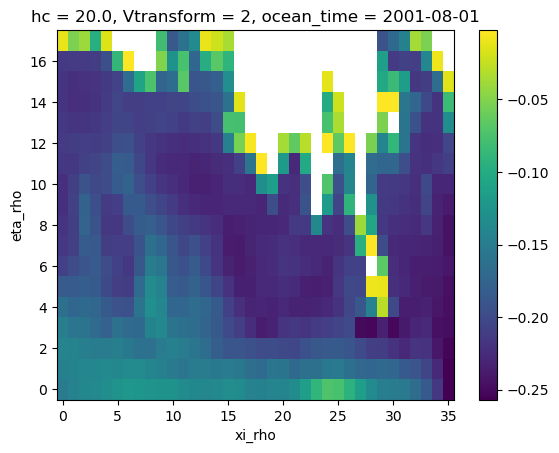### 2. Bilinear example¶

:

regrid_masked3 = xesmf.Regridder(ds, ds_coarse, method="bilinear")
zeta_remapped3.isel(ocean_time=0).plot()

:

<matplotlib.collections.QuadMesh at 0x7f914821c7c0>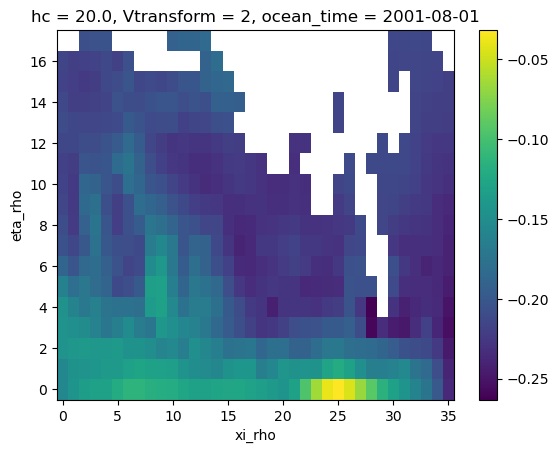## Extrapolation¶

As we saw in the previous example, the bilinear interpolation was not providing a value at all the destination points. This is where the extrapolation becomes useful. xESMF allows to use the ESMF algorithms described in this section of the ESMF documentation. This is a very short example and more options are available. Please refer to the aforementioned documentation for more details.

:

regrid_extrap = xesmf.Regridder(
ds, ds_coarse, method="bilinear", extrap_method="nearest_s2d"
)

:

zeta_remapped_extrap = regrid_extrap(ds["zeta"])

:

zeta_remapped_extrap.isel(ocean_time=0).plot()

:

<matplotlib.collections.QuadMesh at 0x7f9148194820>